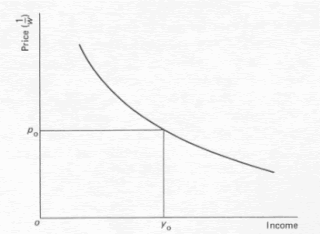## Lionel Robbins The Supply Of Effort

Lionel Robbins The Supply Of Effort

Robbins's essay (1930) on the supply of effort problem is a remarkable example of a standard method to reach a conclusion which should have been obvious in the first place. What is strange is how such great economists as Pigou and Knight should have got the analysis wrong in the first place. Incidentally it should be recalled that their mistake was not the vulgar one of arguing that a tax cut will cause a man to work more but the reverse, namely that he will certainly work less when the net wage rises. The analysis can be set out as follows:

Income equals the wage-rate times hours worked i.e.

Y= W*L

It follows that the percentage change in income equals the percentage change in the wage rate plus the percentage change in hours worked

y =w + 1

where lower-case letters mean 'percentage change in'. The elasticity of demand for income in terms of effort is y/l i.e. y/l=(w/l) + l
If this is greater than unity, w and / have the same sign, i.e. as the wage rate increases so does effort. This is the Robbins formu­lation (see Figure 10.2) which he himself stated was well known and accepted. He then points out that the price of income in terms of effort is the inverse of the wage. The higher the wage the less effort that has to be put in to acquire a unit of income.

Price on the vertical axis in Figure 1 is the effort price of income i.e. 1/W. Robbins then argues that in the 'normal' case the demand for income will vary inversely with price, i.e. the demand curve is downward sloping.

Figure 1If price is p0, income demanded will be y0. What then is p0Y0? This is equal to yj W0 i.e. the number of hours worked. It is, of course, obvious that as p falls pq (in this case y/w) rises if the elasticity of demand is greater than unity, which is the Robbins proposition.

Nowadays this conclusion is arrived at by any first year student using indifference curves. This is illustrated in figure 2.

Figure 2Which demonstration is superior? This is not really an answerable question but it must be noted that the strength of Robbins's approach is first its emphasis on the price of income and secondly on elasticity. The first of these need not be neglected in the indifference curve treatment while the second is not amenable to indifference curve analysis at all. In addition, a benefit of the Robbins exposition is its commonsense nature, namely its emphasis on income being a normal good the demand for which varies inversely with the cost of acquiring it. If his had remained the usual exposition, the tendency to believe that effort must vary directly with the wage rate might not have persisted or remained as influential as it has. The Robbins approach indicates that there is no presumption that tax cuts (expected to endure) will increase the supply of effort. Empirical studies of some subtlety and detail are required, therefore, to ascertain the effects of such cuts and it would be surprising if they indicated a simple and uniform response. It is a great pity that in the fifty years since the article first appeared so few empirical investigations have been undertaken, and the nonsense that Robbins exposed so elegantly remains in the minds of such a wide variety of influential people.